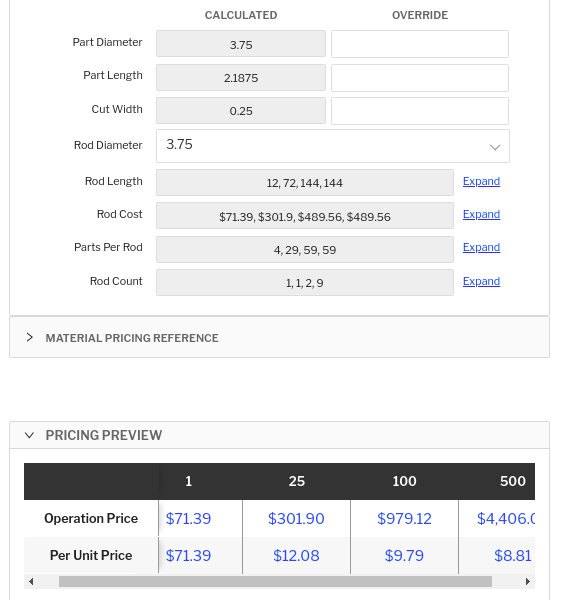# Quantity-specific variables

Quantity specific variables allow you to apply standard variable, table variable, table lookup, and drop down variable logic along individual quantity breaks.

Use quantity specific variables when you want to make overrides along individual quantity breaks and/or when your pricing logic requires different strategies based on the quoted quantity.

In the live pricing UI, a quantity specific variable can be expanded to view/override individual values for each requested quantity. Here is what a standard numeric variable looks like when expanded: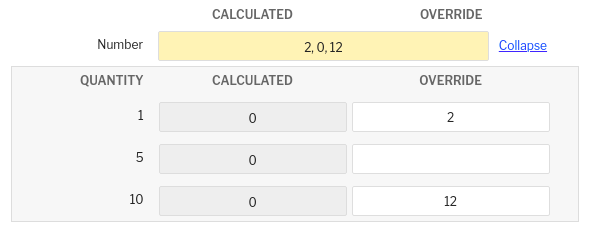The quantities listed on the left correspond to the requested quantities on the quote item. The top row shows comma separated values of overridden or calculated value for each quantity break. The top row will have a yellow color if there is currently an override specified for any quantity.

NOTE: If you have multiple of the same quantity, only one value will be shown to be overridden in the expanded view. You cannot have different override values for quantity specific variables across multiple of the same quantity.

A table variable looks like: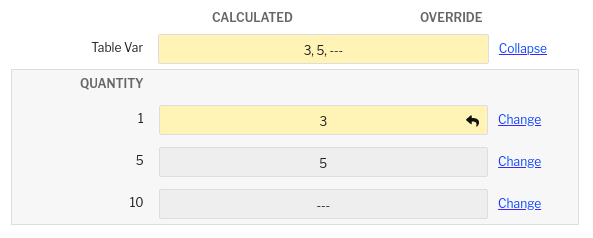Clicking the change button will open a drawer to select a new row of the table to override for the given quantity. Clicking the arrow button will revert the override on the quantity level. A yellow color indicates there is currently an override applied.  Functionality here is the same as it is with quantity-agnostic table variables.

A drop down variable looks like:Clicking the arrow button will revert the override on the quantity level. A yellow color indicates there is currently an override applied. Functionality here is the same as it is with quantity-agnostic drop down variables.

All collapsed together without overrides, the UI looks like this:

###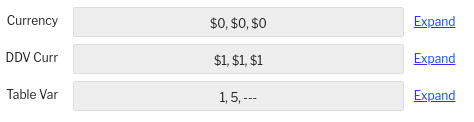P3L Usage

To instantiate a quantity specific variable, use the existing functions ( `var`, `table_var`, `table_lookup`, `drop_down_var`) and specify `quantity_specific=True` as a keyword input:

```std_var = var('Std Var', 1.0, 'Example Standard Variable', number, quantity_specific=True)

row = table_var(
'Table Var',
'Example Table Variable',
'some_custom_table',
create_filter(),
create_order_by(),
'display_column_name',
quantity_specific=True,
)

rows = table_lookup(
'some_custom_table',
create_filter(),
create_order_by(),
quantity_specific=True,
)

ddv = drop_down_var('Drop Down Var', 'a', create_list('a', 'b'), 'Example Drop Down Variable', string, quantity_specific=True)
```

When you specify `quantity_specific=True`, it will return to you an object with all of the same capabilities and properties as a quantity agnostic variable. For example, if you specify `frozen=False` on a `var` or `drop_down_var`, you can perform the same update and option manipulation logic:

```std_var = var('Std Var', 1.0, 'Example Standard Variable', number, frozen=False, quantity_specific=True)
std_var.update(10)
std_var.freeze()

ddv = drop_down_var('Drop Down Var', 'a', create_list('a', 'b'), 'Example Drop Down Variable', string, frozen=False, quantity_specific=True)
ddv.append_option('c')
ddv.sort_options(lambda x: x)
ddv.select_option('b')
```

For table variables and table lookups, we will query the custom table for each quantity. This means you can have quantity-dependent values drive filters on the table:

```row = table_var(
'Table Var',
'Example Table Variable',
'some_custom_table',
create_filter(
filter('numeric_value', '>=', part.qty * 10)
),
create_order_by('numeric_value'),
'display_column_name',
quantity_specific=True,
)
if row:
do_something_quantity_specific = row.numeric_value

rows = table_lookup(
'some_custom_table',
create_filter(
filter('numeric_value', '<=', part.qty + 10)
),
create_order_by(),
quantity_specific=True,
)
do_something_else_quantity_specific = rows.map(lambda x: x.numeric_value)
```

### When to Use

Costing variables are used to represent information that is important for accomplishing logic within your P3L formulas. They are also used as pricing "levers" for the estimator when quoting by allowing the operator to apply overrides. In most situations, costing variables can be thought as "operation" variables. This means that the value the variable contains applies to all quantities being quoted. However in some situations, it is useful to break out costing variables along each quantity break. Use quantity specific variables in this situation.

An example of a classic "operation" variable is something like labor rate. Regardless of how many parts you need to make, your labor rate likely will not change. A variable that might be better as quantity-specific is something like a finish piece price. When reaching out to your finisher with quantities 10, 50, 500, the piece price will likely be lower for the higher quantities. You can then enter these individual piece prices into a quantity-specific variable to calculate a logic-based price for each quantity.

Another variable that might be better to specify along quantity lines is something like material bar length or sheet size.

Let's imagine you need to turn a part that is 5.5 inches long. For quantity 1, you will probably buy one rod that is around 6 inches long. But for quantity 12, you will probably buy one rod that is around 72 inches long. For quantities in the hundreds, you will likely buy several rods of the longest piece of stock the provider sells. Tracking the rod length is an important costing variable to influence your material prep and setup for the machine and bar feeder.

The same analogy works in the sheet metal world. For a high quantity job, you will buy the largest sheet you can find and fit as many parts onto that one sheet. But if you are only making one part, you are going to find a much smaller sheet to cut the part.

### Basic Example - Piece Price for Different Quantities

Let's walk through that first simple piece price example. A customer requests a quote on a simple part with an anodize finish. The customer requests pricing for quantities 10, 50, 200, and 500. You drop the part into Paperless Parts, and share the part with your finish provider. He responds with a flat lot charge cost of \$200 and piece prices of \$4, \$3, \$2, and \$1 respectively. Lets build a simple formula to enable you to input the respective piece prices. The formula would look something like this:

```lot_charge = var('Lot Charge', 0, 'flat lot charge', currency)
piece_price = var('Piece Price', 0, 'cost per piece', currency, quantity_specific=True)

PRICE = lot_charge + piece_price * part.qty
DAYS = 0
```

Then in the live pricing UI, we simply input the values we got from our outside service provider and our final price is calculated: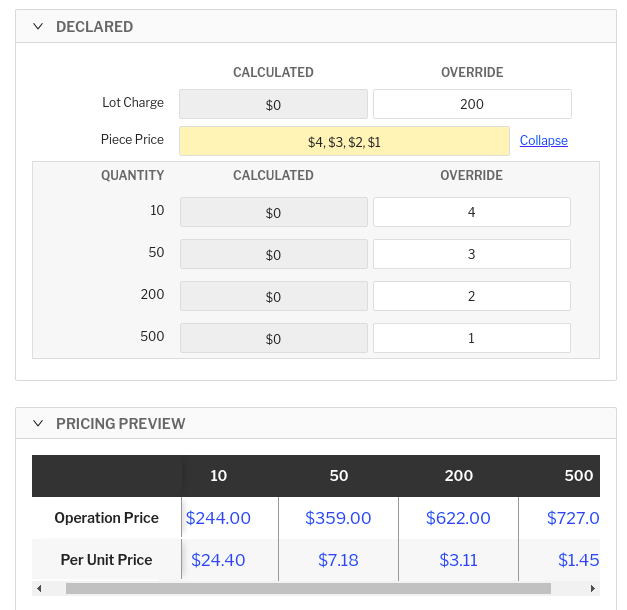### Advanced Example - Rod Selection for Each Quantity w/ Tables

Let's expand on the material rod selection example I mentioned above. Let's set up an operation that allows you to select the most cost effective size rod given a list of rods from a custom table. This is an advanced example that extends/modifies the example discussed in our custom tables article. I recommend reading through that article before working through this example. We will reference the same custom table called material_inventory that looks like this: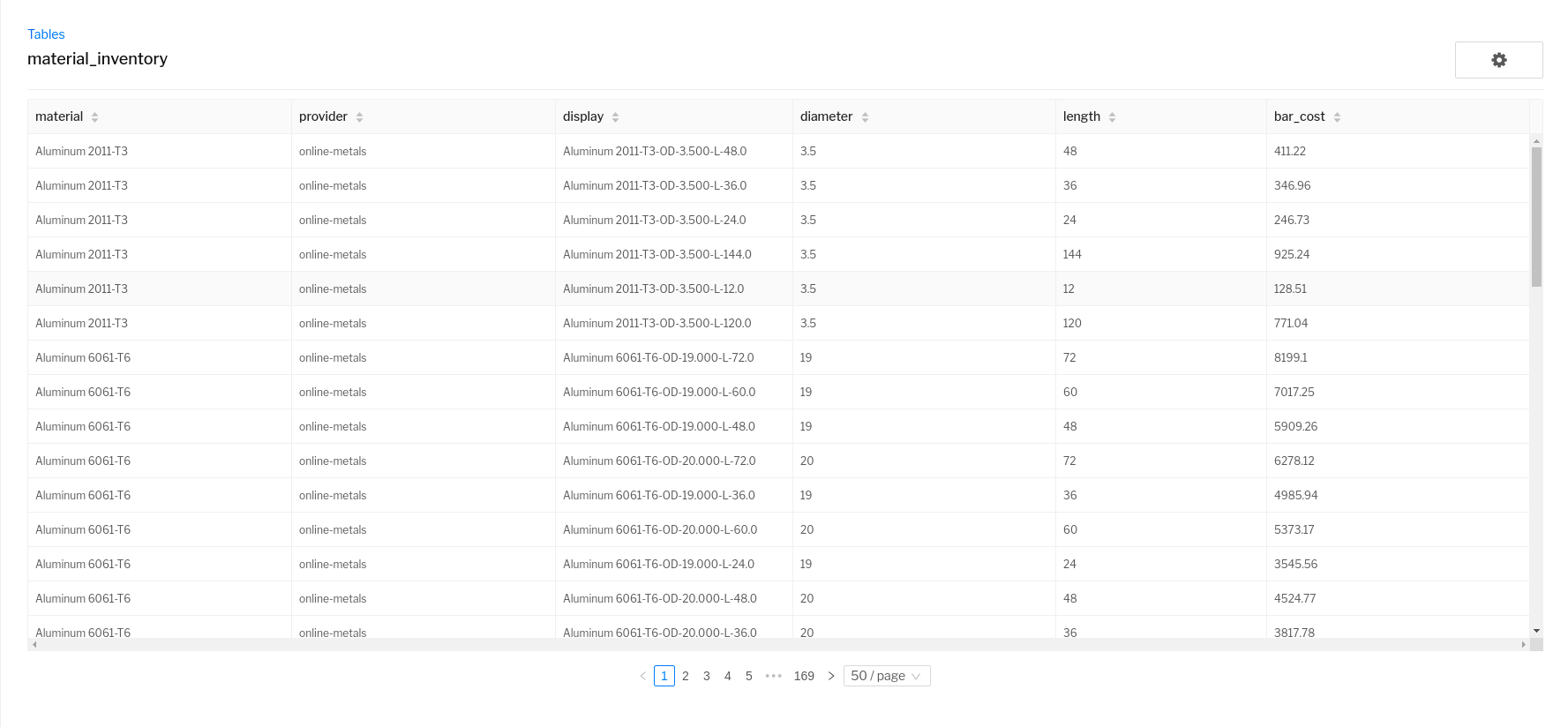The first version of this will utilize a `table_var` lookup to simply line out a rod selection for each quantity break. This will allow the user to override which bar is selected for each individual quantity, but will not do anything smart in terms of automatically selecting the best rod for the job.

```units_in()
lathe = analyze_lathe()

part_diameter = var('Part Diameter', 0, '', number, frozen=False)
part_diameter.freeze()

part_length = var('Part Length', 0, '', number, frozen=False)
part_length.update(lathe.stock_length)
part_length.freeze()

cut_width = var('Cut Width', 0.25, 'width of cut on inches for saw', number)

mat_selection = table_var(
'Material Selection',
'look up for dynamic cylindrical stock selection',
'material_inventory',
create_filter(
filter('material', '=', part.material),
filter('diameter', '>=', part_diameter),
filter('length', '>=', part_length),
),
create_order_by('diameter', '-length', 'bar_cost'),
'display',
quantity_specific=True,
)

rod_length = var('Rod Length', 0, '', number, frozen=False, quantity_specific=True)
rod_cost = var('Rod Cost', 0, '', currency, frozen=False, quantity_specific=True)
if mat_selection:
rod_length.update(mat_selection.length)
rod_cost.update(mat_selection.bar_cost)
rod_length.freeze()
rod_cost.freeze()

parts_per_rod = var('Parts Per Rod', 0, '', number, frozen=False, quantity_specific=True)
parts_per_rod.update(floor(rod_length / (part_length + cut_width)))
parts_per_rod.freeze()

rod_count = var('Rod Count', 0, '', number, frozen=False, quantity_specific=True)
if parts_per_rod:
rod_count.update(ceil(part.qty / parts_per_rod))
rod_count.freeze()

PRICE = rod_count * rod_cost
DAYS = 0

```

Note that once we establish a quantity specific variable, all downstream variables that depend upon resources from that variable must also be quantity-specific.

Here is what that operation looks like in the live pricing UI for quantities 1, 25, 100, and 500: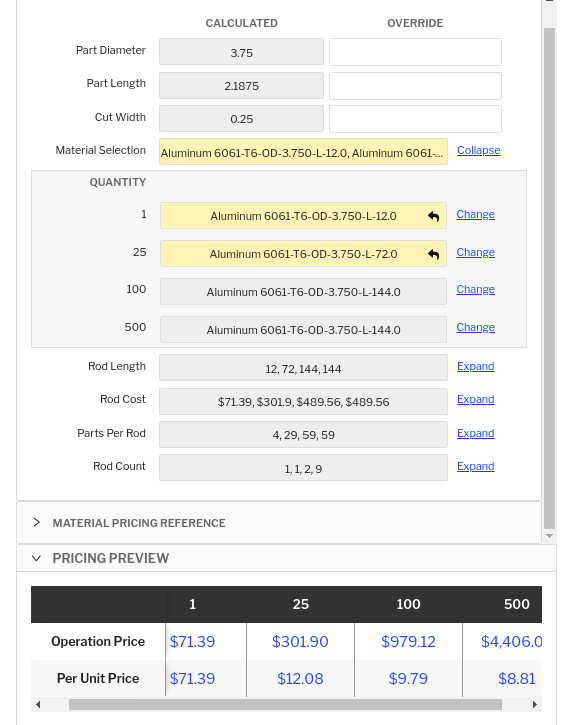We expanded out the "Material Selection" table variable and applied an override for the first two quantities. The shortest rods that satisfy the quantity were a 12 inch and 72 inch rod respectively. Then downstream variables dynamically updated from referencing the overriden table variable, such as rod length, rod cast, parts per rod, and rod count.

Let's extend that example to automatically select the best rod length for each quantity break. We need to implement logic that will automatically associate the 12 inch and 72 inch rod for the "Material Selection" table variable. We will achieve this by using a dynamic `table_var` by specifying `frozen=False`. This will allow us to specify the specific row of the table we want and not force it to be the first row in accordance to the `order_by` conditions. The operation code below will query the custom table in the exact same way, and then filter the rows returned down to only those that share the smallest diameter amongst those rows. We will then select from these rows the shortest and most cost effective bar for each quantity. This logic is implemented by checking to see if any bar length can singularly accomplish the entire quantity. Here is the updated operation code:

```units_in()
lathe = analyze_lathe()

part_diameter = var('Part Diameter', 0, '', number, frozen=False)
part_diameter.freeze()

part_length = var('Part Length', 0, '', number, frozen=False)
part_length.update(lathe.stock_length)
part_length.freeze()

cut_width = var('Cut Width', 0.25, 'width of cut on inches for saw', number)

mat_selection = table_var(
'Material Selection',
'look up for dynamic cylindrical stock selection',
'material_inventory',
create_filter(
filter('material', '=', part.material),
filter('diameter', '>=', part_diameter),
filter('length', '>=', part_length),
),
create_order_by('diameter', '-length', 'bar_cost'),
'display',
frozen=False,
quantity_specific=True,
)

# collect smallest diameter of rods that can satisfy the part diameter
# as the value to restrict our search
if mat_selection.rows:
diameters = mat_selection.rows.map(lambda x: x.diameter)
# collect unique diameters
unique_diameters = create_list()
for d in iterate(diameters):
if d not in unique_diameters:
unique_diameters.append(d)
else:
unique_diameters = create_list(0)

min_diameter = min(unique_diameters)
min_diameter_rows = mat_selection.rows.filter(lambda x: x.diameter == min_diameter)
if min_diameter_rows:
# select bar length based on satisfying quantities most effectively
# if a bar can satisfy the entire quantity, select it as the option
min_diameter_rows.sort(lambda x: create_multi_sort(x.length, x.bar_cost))
use_row = None
equivalent_length = part_length + cut_width
for rod_option in iterate(min_diameter_rows):
if rod_option.length >= part.qty * equivalent_length:
use_row = rod_option
break
if not use_row:
# select the longest and cheapest rod
use_row = min_diameter_rows.sort(lambda x: create_multi_sort(-x.length, x.bar_cost))
mat_selection.select_by_row(use_row)
else:
# select first entry to freeze variable
mat_selection.select_first()

rod_length = var('Rod Length', 0, '', number, frozen=False, quantity_specific=True)
rod_cost = var('Rod Cost', 0, '', currency, frozen=False, quantity_specific=True)
if mat_selection.value:
rod_length.update(mat_selection.value.length)
rod_cost.update(mat_selection.value.bar_cost)
rod_length.freeze()
rod_cost.freeze()

parts_per_rod = var('Parts Per Rod', 0, '', number, frozen=False, quantity_specific=True)
parts_per_rod.update(floor(rod_length / (part_length + cut_width)))
parts_per_rod.freeze()

rod_count = var('Rod Count', 0, '', number, frozen=False, quantity_specific=True)
if parts_per_rod:
rod_count.update(ceil(part.qty / parts_per_rod))
rod_count.freeze()

PRICE = rod_count * rod_cost
DAYS = 0

```

And here we can see correctly that the most optimal rods are automatically selected in the UI without needing to be overriden: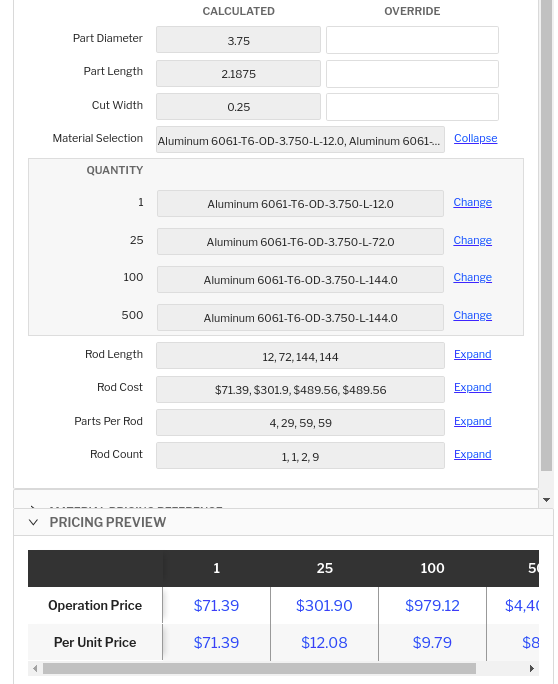Is is important to note that when using quantity specific `table_var` instances, a new query will be performed on the table for each quantity. This will require more computation for the system to do, and thus make price recalculation a bit slower. The above operation code is effective in the fact that it can automatically determine the best rod length and it allows the user to explicitly override selection for each quantity, but the query to the table itself actually does not have to be done for each quantity since the filter conditions are not dependent on the quantity. A better way to accomplish this logic is to use a quantity-agnostic `table_lookup` and filter through the rows of the lookup to find the best rod option. The following operation code demonstrates how to accomplish this logic. Notice in this operation we actually collect all of the diameter options into a drop down variable the user can select from, defaulting to the smallest diameter.

```units_in()
lathe = analyze_lathe()

part_diameter = var('Part Diameter', 0, '', number, frozen=False)
part_diameter.freeze()

part_length = var('Part Length', 0, '', number, frozen=False)
part_length.update(lathe.stock_length)
part_length.freeze()

cut_width = var('Cut Width', 0.25, 'width of cut on inches for saw', number)

rows = table_lookup(
'material_inventory',
create_filter(
filter('material', '=', part.material),
filter('diameter', '>=', part_diameter),
filter('length', '>=', part_length),
),
create_order_by('diameter', '-length', 'bar_cost'),
)

# collect smallest diameter of rods that can satisfy the part diameter
# as the value to restrict our search
search_diameter = drop_down_var('Rod Diameter', 0, create_list(), '', number, frozen=False)
if rows:
diameters = rows.copy().map(lambda x: x.diameter)
# collect unique diameters
unique_diameters = create_list()
for d in iterate(diameters):
if d not in unique_diameters:
unique_diameters.append(d)

# select smallest diameter, should be first entry as rows are already sorted
search_diameter.update_options(unique_diameters)
search_diameter.select_option_or_default(min(unique_diameters))
else:
search_diameter.select_default()

min_diameter_rows = rows.copy().filter(lambda x: x.diameter == search_diameter.value)
use_row = None
if min_diameter_rows:
# select bar length based on satisfying quantities most effectively
min_diameter_rows.sort(lambda x: create_multi_sort(x.length, x.bar_cost))
use_row = None
equivalent_length = part_length + cut_width
for rod_option in iterate(min_diameter_rows):
if rod_option.length >= part.qty * equivalent_length:
use_row = rod_option
break
if not use_row:
# select the longest and cheapest rod
use_row = min_diameter_rows.sort(lambda x: create_multi_sort(-x.length, x.bar_cost))

rod_length = var('Rod Length', 0, '', number, frozen=False, quantity_specific=True)
rod_cost = var('Rod Cost', 0, '', currency, frozen=False, quantity_specific=True)
if use_row:
rod_length.update(use_row.length)
rod_cost.update(use_row.bar_cost)
rod_length.freeze()
rod_cost.freeze()

parts_per_rod = var('Parts Per Rod', 0, '', number, frozen=False, quantity_specific=True)
parts_per_rod.update(floor(rod_length / (part_length + cut_width)))
parts_per_rod.freeze()

rod_count = var('Rod Count', 0, '', number, frozen=False, quantity_specific=True)
if parts_per_rod:
rod_count.update(ceil(part.qty / parts_per_rod))
rod_count.freeze()

PRICE = rod_count * rod_cost
DAYS = 0

```

Here is what the live pricing UI looks like from this operation code: